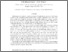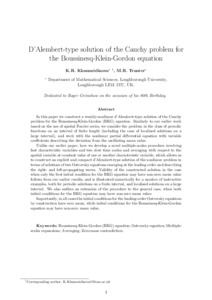# D'Alembert‐type solution of the Cauchy problem for the Boussinesq‐Klein‐Gordon equation

Khusnutdinova, K.R. and , 2019. D'Alembert‐type solution of the Cauchy problem for the Boussinesq‐Klein‐Gordon equation. Studies in Applied Mathematics, 142 (4), pp. 551-585. ISSN 0022-2526Preview
Text
14171_a1568_Tranter.pdf - Post-print

## Abstract

In this paper, we construct a weakly‐nonlinear d'Alembert‐type solution of the Cauchy problem for the Boussinesq‐Klein‐Gordon (BKG) equation. Similarly to our earlier work based on the use of spatial Fourier series, we consider the problem in the class of periodic functions on an interval of finite length (including the case of localized solutions on a large interval), and work with the nonlinear partial differential equation with variable coefficients describing the deviation from the oscillating mean value. Unlike our earlier paper, here we develop a novel multiple‐scales procedure involving fast characteristic variables and two slow time scales and averaging with respect to the spatial variable at a constant value of one or another characteristic variable, which allows us to construct an explicit and compact d'Alembert‐type solution of the nonlinear problem in terms of solutions of two Ostrovsky equations emerging at the leading order and describing the right‐ and left‐propagating waves. Validity of the constructed solution in the case when only the first initial condition for the BKG equation may have nonzero mean value follows from our earlier results, and is illustrated numerically for a number of instructive examples, both for periodic solutions on a finite interval, and localized solutions on a large interval. We also outline an extension of the procedure to the general case, when both initial conditions may have nonzero mean values. Importantly, in all cases, the initial conditions for the leading‐order Ostrovsky equations by construction have zero mean, while initial conditions for the BKG equation may have nonzero mean values.

Item Type: Journal article
Publication Title: Studies in Applied Mathematics
Creators: Khusnutdinova, K.R. and Tranter, M.R.
Publisher: Wiley-Blackwell
Date: May 2019
Volume: 142
Number: 4
ISSN: 0022-2526
Identifiers:
NumberType
10.1111/sapm.12263DOI
Divisions: Schools > School of Science and Technology
Record created by: Jonathan Gallacher
Date Added: 28 Jun 2019 12:43Edit View